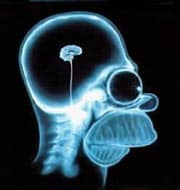# Science without sense…double nonsense

## Even a blind donkey…

This post is also available in: Spanish

…finds the well sometimes. It is not surprising. Most often, the donkey is not able to find the well, but if it tries it many times, it can ever end up finding the well at last, if only by chance.

Actually, we use this saying to refer to the fact that if we repeat an action too insistently we can end up having some mishap.

For example, let’s make a parallel between the donkey’s going to the well and doing a hypothesis testing. Do you think they have nothing to do? Well, they have: if we do hypothesis testing insistently we can finish getting a drawback, it will be no other than committing a type I error. Let me explain before you think I’ve hit my head with the edge of the well.

Remember that whenever we do a hypothesis testing we set a null hypothesis (H0) that says that the observed difference between comparison groups is due to chance. Then we calculate the probability that the difference is due to chance and, if less than a certain value (typically 0.05), we reject H0 and affirm that it’s highly unlikely that the difference is due to chance, therefore considering it real. But, of course, highly unlikely doesn’t mean certain. There’s always a 5% chance of rejecting H0 being true, therefore assuming an effect as real when it doesn’t actually exist. This is what it’s called making a type I error.

If we make multiple comparisons, the probability of making an error increases. For example, if we make 100 comparisons, we’ll expect to be mistaken about five times because the likelihood of committing error every time will be 5% (and the probability of being right, 95%).

So we ask ourselves, if we do n comparisons, what is the probability of having at least one false positive? This is a little laborious to calculate, because we’d have to calculate the probability of 1,2, …, n-1 and n false positives using binomial probability. So we resorted to a trick often used in the calculation of probabilities, which is to calculate the probability of the complementary event. Let me explain. The probability of at least one false positive plus the probability of no false positives will be equal to one (100%). Then, the probability of at least one false positive is equal to 1 minus the probability of none.

And what is the probability of none? We’ve already said that the probability of being right in every contrast is 0.95. The probability of no committing mistakes in n contrast will be 0.95n. So, the probability of getting at least one false positive is 1-0.95n.

Imagine we do 20 comparisons. The probability of making at least one type I error will be 1-0.9520 = 0.64. There will be a 64% chance of committing an error and, just by chance, take the effect as real when it really doesn’t exist.

Well, what nonsense, you will say. Who is going to do so many comparisons knowing the dangers it has? But, if you think about it for a while, you have seen it many times. Who has not read an article about a trial involving a post hoc multiple comparisons study? It is quite common when the trial does not yield results with statistical significance. The authors tend to squeeze and torturing the data until they find a satisfactory outcome.

However, always distrust of post hoc studies. The trial should try to answer a question previously established and not to seek answers to questions that we can propose after ending the trial, dividing participants into groups based on characteristics that have nothing to do with the initial randomization.

Anyway, as it is a hard habit to eradicate, we can indeed require trial authors to have a number of precautions if they want to do post hoc studies with multiple hypothesis testing. First, any analysis is done with the trial results must be specified when the trial is planned and not once over. Second, groups must have some biological plausibility. Third, you should avoid making multiple comparisons with subgroups if the overall trial results are not significant. And finally, always use a technique to keep the probability of type I error below 5%, as the Bonferroni’s correction or otherwise.

There’s one last advice for us: to evaluate carefully the differences to be found among the different subgroups, particularly when p values are not very small, between 0.01 and 0.05.

And here we leave the post hoc studies and their traps. We have not said that there are more examples of multiple post-randomization comparisons in addition to analyzing subgroups. I think of the example of the cohort studies studying different effects produced by the same exposure, or of intermediate analysis made during sequential trials to see if the ending rule is met. But that is another story…

This site uses Akismet to reduce spam. Learn how your comment data is processed.